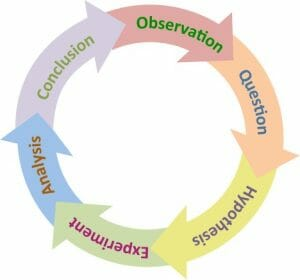# Scientific Method

### August 31, 2019

The scientific method is a series of processes that people can use to gather knowledge about the world around them, improve that knowledge, and, through gaining knowledge, attempt to explain why and/or how things occur. This method involves making observations, forming questions, making hypotheses, doing an experiment, analyzing the data, and forming a conclusion. Every scientific experiment performed is an example of the scientific method in action, but it is also used by non-scientists in everyday situations.

### Scientific Method Steps

The exact steps of the scientific method vary from source to source, but the general procedure is the same: acquiring knowledge through observation and testing.#### 1.Making an Observation

The first step of the scientific method is to make an observation about the world around you. The scientific method is used when one does not know why/how something is occurring and wants to uncover the answer, but before one can even question an occurrence, they must notice something puzzling in the first place.

Next, one must ask a question based on their observations, such as why/how is this thing occurring? Why/how does it happen this way?

#### 3.Forming a Hypothesis

A hypothesis is an educated guess to explain the phenomena occurring based on prior observations. It answers the question posed in the previous step. Hypotheses can be specific or more general depending on the question being asked, but all hypotheses must be testable by gathering evidence that can be measured. If a hypothesis is not testable, then it is impossible to perform an experiment to determine whether the hypothesis is supported by evidence.

#### 4.Performing an Experiment

After forming a hypothesis, an experiment must be set up and performed to test the hypothesis. An experiment must have an independent variable, which is something that is manipulated by the person doing the experiment, and a dependent variable, which is the thing being measured (and which may be affected by the independent variable). All other variables must be controlled so that they do not affect the outcome. During an experiment, data is collected.

#### 5.Analyzing Data

After performing an experiment and collecting data, one must analyze the data. Research experiments are usually analyzed with statistical software in order to determine relationships among the data.

#### 6.Forming a Conclusion

The last step of the scientific method is to form a conclusion. If the data support the hypothesis, then the hypothesis may be the explanation for the phenomena. When a conclusion is drawn, the research can be presented to others to inform them of the findings and receive input about the validity of the conclusion drawn from the research.FB Page

@ELITE_Mv